### LU Decomposition for solving linear equations in C++

Let A be a square matrix. An LU factorization refers to the factorization of A, with proper row and/or column orderings or permutations, into two factors, a lower triangular matrix L and an upper triangular matrix U,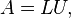$A = LU, \,$
In the lower triangular matrix all elements above the diagonal are zero, in the upper triangular matrix, all the elements below the diagonal are zero. For example, for a 3-by-3 matrix A, its LU decomposition looks like this: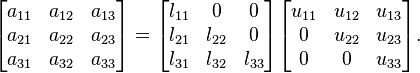$\begin{bmatrix} a_{11} & a_{12} & a_{13} \\ a_{21} & a_{22} & a_{23} \\ a_{31} & a_{32} & a_{33} \\ \end{bmatrix} = \begin{bmatrix} l_{11} & 0 & 0 \\ l_{21} & l_{22} & 0 \\ l_{31} & l_{32} & l_{33} \\ \end{bmatrix} \begin{bmatrix} u_{11} & u_{12} & u_{13} \\ 0 & u_{22} & u_{23} \\ 0 & 0 & u_{33} \\ \end{bmatrix}.$
Without a proper ordering or permutations in the matrix, the factorization may fail to materialize. For example, it is easy to verify (by expanding the matrix multiplication) that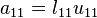$a_{11} = l_{11} u_{11}$. If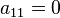$a_{11} = 0$, then at least one of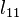$l_{11}$ and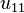$u_{11}$ has to be zero, which implies either L or U is singular. This is impossible if A is nonsingular. This is a procedural problem. It can be removed by simply reordering the rows of A so that the first element of the permuted matrix is nonzero. The same problem in subsequent factorization steps can be removed the same way, see the basic procedure below.
It turns out that a proper permutation in rows (or columns) is sufficient for the LU factorization. The LU factorization with Partial Pivoting refers often to the LU factorization with row permutations only,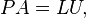$PA = LU, \,$
where L and U are again lower and upper triangular matrices, and P is a permutation matrix which, when left-multiplied to A, reorders the rows of A. It turns out that all square matrices can be factorized in this form, and the factorization is numerically stable in practice. This makes LUP decomposition a useful technique in practice.
An LU factorization with full pivoting involves both row and column permutations,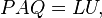$PAQ = LU, \,$
where LU and P are defined as before, and Q is a permutation matrix that reorders the columns of A.
An LDU decomposition is a decomposition of the form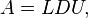$A = LDU, \,$
where D is a diagonal matrix and L and U are unit triangular matrices, meaning that all the entries on the diagonals of L and U are one.
Above we required that A be a square matrix, but these decompositions can all be generalized to rectangular matrices as well. In that case, L and P are square matrices which each have the same number of rows as A, while U is exactly the same shape as AUpper triangular should be interpreted as having only zero entries below the main diagonal, which starts at the upper left corner.

### <![CDATA[ #include "linearEquation.h" #include <iostream> #include <stdio.h> #include <Windows.h> using namespace std; #define size 10 void read_elements(float mat[size][size], float vect[size], int n){ cout<<"Enter all coefficients of matrix : "; for(int i=1;i<=n;i++) { cout<<"\nRow "<<i<<"\n "; for(int j=1;j<=n;j++) cin>>mat[i][j]; } cout<<"Enter elements of b matrix"<<endl; for(int i=1;i<=n;i++) cin>>vect[i]; } void LU_Decomp(float A[size][size], float L[size][size], float U[size][size], int n){ //***** COMPLETE THIS FUNCTION TO DECOMPOSE THE MATRIX A INTO L and U *********// for(int k=1;k<=n;k++){ for(int j=k;j<=n;j++){ float cc=0; for(int t=1;t<=k-1;t++){ cc=cc+L[k][t]*U[t][j]; } U[k][j]=A[k][j]-cc; } L[k][k]=1; for(int i=k+1;i<=n;i++){ float bb=0; for(int t=1;t<=k-1;t++){ bb=bb+L[i][t]*U[t][k]; } L[i][k]=(A[i][k]-bb)/U[k][k]; } } } void show_LU(float L[size][size],float U[size][size], int n){ //***** Displaying LU matrix*******// cout<<endl<<endl<<"L matrix is "<<endl; for(int i=1;i<=n;i++) { for(int j=1;j<=n;j++) cout<<L[i][j]<<" \t "; cout<<endl; } cout<<endl; cout<<endl<<endl<<"U matrix is "<<endl; for(int i=1;i<=n;i++) { for(int j=1;j<=n;j++) cout<<U[i][j]<<" \t"; cout<<endl; } } void solve_Lyb(float L[size][size], float y[size], float b[size], int n){ //***** COMPLETE THIS FUNCTION TO SOLVE Ly=b (forward-subtitution method) *********// y=b/L; for(int i=2;i<=n;i++){ int ll=0; for(int j=1;j<=i-1;j++){ ll=ll+L[i][j]*y[j]; } y[i]=(b[i]-ll)/L[i][i]; } } void solve_Uxy(float U[size][size], float x[size], float y[size], int n){ //***** COMPLETE THIS FUNCTION TO SOLVE Ux=y (back-substitution method) *********// x[n]=y[n]/U[n][n]; for(int i=n-1;i>=1;i--){ float uu=0; for(int j=i+1;j<=n;j++){ uu=uu+U[i][j]*x[j]; } x[i]=(y[i]-uu)/U[i][i]; } } void show_results(float x[size], int n){ //******** DISPLAYING FINAL RESULTS *********// cout<<endl<<"Set of result is : "<<endl; for(int i=1;i<=n;i++) cout<<"x["<<i<<"] = "<<x[i]<<endl; } void main(){ cout<<"++++++++++++++++ Start Q2(a) Answer ++++++++++++++++++++++++"<<endl; int n1; float A1[size][size],x1[size]={0},b1[size]; cout<<"Enter the order of matrix [ <= 10 ]: "<<endl; cin>>n1; cout<<"Enter the uper triangular matrix elements "<<endl; read_elements(A1,b1,n1); solve_Uxy(A1,x1,b1,n1); show_results(x1, n1); cout<<"++++++++++++++++ End Q2(a) Answer ++++++++++++++++++++++++"<<endl; cout<<"++++++++++++++++ Start Q2(b) Answer ++++++++++++++++++++++++"<<endl; int n2; float A2[size][size],x2[size]={0},b2[size]; cout<<"Enter the order of matrix [ <= 10 ]: "<<endl; cin>>n2; cout<<"Enter the lower triangular matrix elements "<<endl; read_elements(A2,b2,n2); solve_Lyb(A2,x2,b2,n2); show_results(x2, n2); cout<<"++++++++++++++++ End Q2(b) Answer ++++++++++++++++++++++++"<<endl; /*Q4*/ cout<<"++++++++++++++++ Start Q4 Answer ++++++++++++++++++++++++"<<endl; int n; float A[size][size],x[size]={0},b[size],L[size][size]={0},U[size][size]={0},y[size]={0}; cout<<"Enter the order of matrix [ <= 10 ]: "; cin>>n; read_elements(A,b,n); //********** LU decomposition *****// LU_Decomp(A,L,U,n); //******** Displaying LU matrix**********// show_LU(L,U,n); //***** FINDING y; Ly=b; (forward subtitution method) *********// solve_Lyb(L,y,b,n); //********** FINDING x; Ux=y***********// solve_Uxy(U,x,y,n); //*********** DISPLAYING RESULTS**************// show_results(x, n); cout<<"++++++++++++++++ End Q4 Answer ++++++++++++++++++++++++"<<endl; system("PAUSE"); } ]]> Output of this program

/* OUTPUT OF MAIN METHODE */

++++++++++++++++ Start Q2(a) Answer ++++++++++++++++++++++++
Enter the order of matrix [ <= 10 ]:
3
Enter the uper triangular matrix elements
Enter all coefficients of matrix :
Row 1
2
3
4

Row 2
0
2
8

Row 3
0
0
3
Enter elements of b matrix
16
18
18

Set of result is :
x = 18.5
x = -15
x = 6
++++++++++++++++ End Q2(a) Answer ++++++++++++++++++++++++

++++++++++++++++ Start Q2(b) Answer ++++++++++++++++++++++++
Enter the order of matrix [ <= 10 ]:
3
Enter the lower triangular matrix elements
Enter all coefficients of matrix :
Row 1
2
0
0

Row 2
3
4
0

Row 3
2
3
5
Enter elements of b matrix
18
16
20

Set of result is :
x = 9
x = -2.75
x = 2.2
++++++++++++++++ End Q2(b) Answer ++++++++++++++++++++++++

++++++++++++++++ Start Q4 Answer +++++++++++++++++++++++
Enter the order of matrix [ <= 10 ]: 4
Enter all coefficients of matrix :
Row 1
2
1
-1
2

Row 2
4
5
-3
6

Row 3
-2
5
-2
6

Row 4
4
11
-4
8
Enter elements of b matrix
5
9
4
2

L matrix is
1        0       0       0
2        1       0       0
-1       2       1       0
2        3       -1      1

U matrix is
2       1       -1      2
0       3       -1      2
0       0       -1      4
0       0       0       2

Set of result is :
x = 1
x = -2
x = 1
x = 3
++++++++++++++++ End Q4 Answer ++++++++++++++++++++++++
Press any key to continue . . .